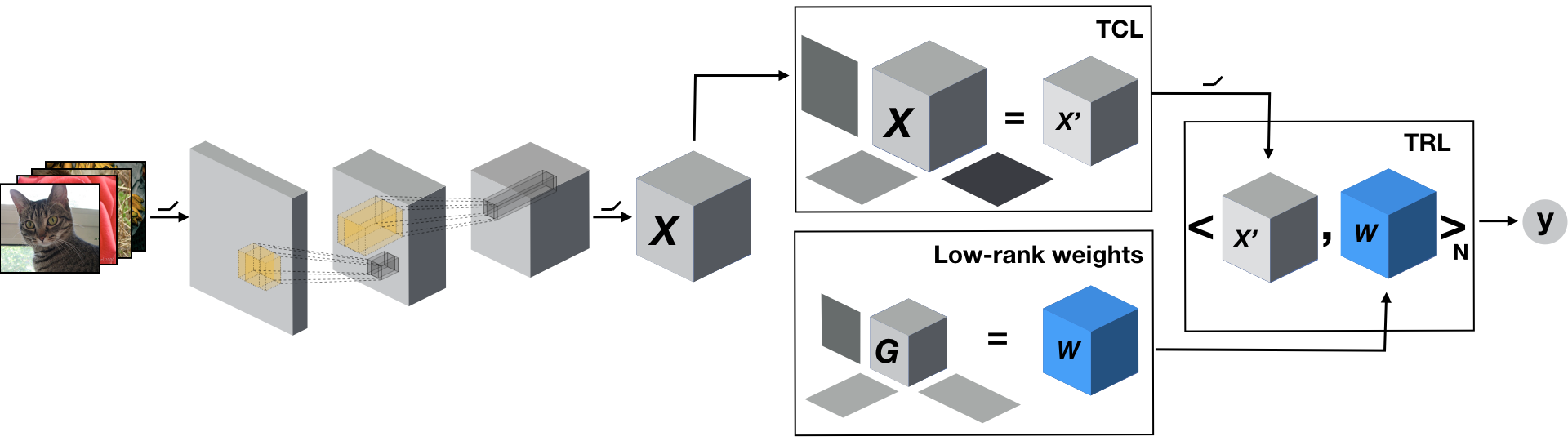# Tensor Regression Layers

In deep neural networks, while convolutional layers map between high-order activation tensors, the output is still obtained through linear regression: first the activation is flattened before being passed through linear layers.

This approach has several drawbacks:

• Linear regression discards topological (e.g. spatial) information.

• Very large number of parameters (product of the dimensions of the input tensor times the size of the output)

• Lack of robustness

A Tensor Regression Layer (TRL) generalizes the concept of linear regression to higher-order but alleviates the above issues. It allows to preserve and leverage multi-linear structure while being parsimonious in terms of number of parameters. The low-rank constraints also acts as an implicit reguralization on the model, typically leading to better sample efficiency and robustness.## TRL in TensorLy-Torch

Now, let’s see how to do this in code with TensorLy-Torch

## Random TRL

Let’s first see how to create and train a TRL from scratch

```import tltorch
import torch
from torch import nn
import numpy as np
```
```input_shape = (4, 5)
output_shape = (6, 2)
batch_size = 2

device = 'cpu'

x = torch.randn((batch_size,) + input_shape,
dtype=torch.float32, device=device)
```
```trl = tltorch.TRL(input_shape, output_shape, rank='same')
```
```result = trl(x)
```